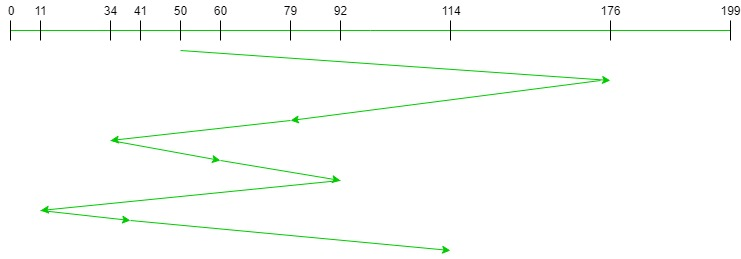# FCFS Disk Scheduling Algorithms

• Difficulty Level : Medium
• Last Updated : 28 May, 2021

Prerequisite: Disk scheduling algorithms.
Given an array of disk track numbers and initial head position, our task is to find the total number of seek operations done to access all the requested tracks if First Come First Serve (FCFS) disk scheduling algorithm is used.

First Come First Serve (FCFS)
FCFS is the simplest disk scheduling algorithm. As the name suggests, this algorithm entertains requests in the order they arrive in the disk queue. The algorithm looks very fair and there is no starvation (all requests are serviced sequentially) but generally, it does not provide the fastest service.

Algorithm:

1. Let Request array represents an array storing indexes of tracks that have been requested in ascending order of their time of arrival. ‘head’ is the position of disk head.
2. Let us one by one take the tracks in default order and calculate the absolute distance of the track from the head.
3. Increment the total seek count with this distance.
4. Currently serviced track position now becomes the new head position.
5. Go to step 2 until all tracks in request array have not been serviced.

Example:

```Input:
Request sequence = {176, 79, 34, 60, 92, 11, 41, 114}

Output:
Total number of seek operations = 510
Seek Sequence is
176
79
34
60
92
11
41
114```

The following chart shows the sequence in which requested tracks are serviced using FCFS.Therefore, the total seek count is calculated as:

```= (176-50)+(176-79)+(79-34)+(60-34)+(92-60)+(92-11)+(41-11)+(114-41)
= 510```

Implementation:
Implementation of FCFS is given below. Note that distance is used to store absolute distance between head and current track position.

## C++

 `// C++ program to demonstrate``// FCFS Disk Scheduling algorithm` `#include ``using` `namespace` `std;` `int` `size = 8;` `void` `FCFS(``int` `arr[], ``int` `head)``{``    ``int` `seek_count = 0;``    ``int` `distance, cur_track;` `    ``for` `(``int` `i = 0; i < size; i++) {``        ``cur_track = arr[i];` `        ``// calculate absolute distance``        ``distance = ``abs``(cur_track - head);` `        ``// increase the total count``        ``seek_count += distance;` `        ``// accessed track is now new head``        ``head = cur_track;``    ``}` `    ``cout << ``"Total number of seek operations = "``         ``<< seek_count << endl;` `    ``// Seek sequence would be the same``    ``// as request array sequence``    ``cout << ``"Seek Sequence is"` `<< endl;` `    ``for` `(``int` `i = 0; i < size; i++) {``        ``cout << arr[i] << endl;``    ``}``}` `// Driver code``int` `main()``{` `    ``// request array``    ``int` `arr[size] = { 176, 79, 34, 60, 92, 11, 41, 114 };``    ``int` `head = 50;` `    ``FCFS(arr, head);` `    ``return` `0;``}`

## Java

 `// Java program to demonstrate``// FCFS Disk Scheduling algorithm``class` `GFG``{``static` `int` `size = ``8``;` `static` `void` `FCFS(``int` `arr[], ``int` `head)``{``    ``int` `seek_count = ``0``;``    ``int` `distance, cur_track;` `    ``for` `(``int` `i = ``0``; i < size; i++)``    ``{``        ``cur_track = arr[i];` `        ``// calculate absolute distance``        ``distance = Math.abs(cur_track - head);` `        ``// increase the total count``        ``seek_count += distance;` `        ``// accessed track is now new head``        ``head = cur_track;``    ``}` `    ``System.out.println(``"Total number of "` `+``                       ``"seek operations = "` `+``                        ``seek_count);` `    ``// Seek sequence would be the same``    ``// as request array sequence``    ``System.out.println(``"Seek Sequence is"``);` `    ``for` `(``int` `i = ``0``; i < size; i++)``    ``{``        ``System.out.println(arr[i]);``    ``}``}` `// Driver code``public` `static` `void` `main(String[] args)``{``    ``// request array``    ``int` `arr[] = { ``176``, ``79``, ``34``, ``60``,``                  ``92``, ``11``, ``41``, ``114` `};``    ``int` `head = ``50``;` `    ``FCFS(arr, head);``}``}` `// This code is contributed by 29AjayKumar`

## Python3

 `# Python program to demonstrate``# FCFS Disk Scheduling algorithm` `size ``=` `8``;` `def` `FCFS(arr, head):` `    ``seek_count ``=` `0``;``    ``distance, cur_track ``=` `0``, ``0``;` `    ``for` `i ``in` `range``(size):``        ``cur_track ``=` `arr[i];` `        ``# calculate absolute distance``        ``distance ``=` `abs``(cur_track ``-` `head);` `        ``# increase the total count``        ``seek_count ``+``=` `distance;` `        ``# accessed track is now new head``        ``head ``=` `cur_track;``    ` `    ``print``(``"Total number of seek operations = "``,``                                   ``seek_count);` `    ``# Seek sequence would be the same``    ``# as request array sequence``    ``print``(``"Seek Sequence is"``);` `    ``for` `i ``in` `range``(size):``        ``print``(arr[i]);``    ` `# Driver code``if` `__name__ ``=``=` `'__main__'``:` `    ``# request array``    ``arr ``=` `[ ``176``, ``79``, ``34``, ``60``,``            ``92``, ``11``, ``41``, ``114` `];``    ``head ``=` `50``;` `    ``FCFS(arr, head);` `# This code contributed by Rajput-Ji`

## C#

 `// C# program to demonstrate``// FCFS Disk Scheduling algorithm``using` `System;` `class` `GFG``{``static` `int` `size = 8;` `static` `void` `FCFS(``int` `[]arr, ``int` `head)``{``    ``int` `seek_count = 0;``    ``int` `distance, cur_track;` `    ``for` `(``int` `i = 0; i < size; i++)``    ``{``        ``cur_track = arr[i];` `        ``// calculate absolute distance``        ``distance = Math.Abs(cur_track - head);` `        ``// increase the total count``        ``seek_count += distance;` `        ``// accessed track is now new head``        ``head = cur_track;``    ``}` `    ``Console.WriteLine(``"Total number of "` `+``                    ``"seek operations = "` `+``                              ``seek_count);` `    ``// Seek sequence would be the same``    ``// as request array sequence``    ``Console.WriteLine(``"Seek Sequence is"``);` `    ``for` `(``int` `i = 0; i < size; i++)``    ``{``        ``Console.WriteLine(arr[i]);``    ``}``}` `// Driver code``public` `static` `void` `Main(String[] args)``{``    ``// request array``    ``int` `[]arr = { 176, 79, 34, 60,``                  ``92, 11, 41, 114 };``    ``int` `head = 50;` `    ``FCFS(arr, head);``}``}` `// This code is contributed by PrinciRaj1992`

## Javascript

 ``

Output:

```Total number of seek operations = 510
Seek Sequence is
176
79
34
60
92
11
41
114```

My Personal Notes arrow_drop_up